# Using the `FoReco` package for cross-sectional, temporal and cross-temporal point forecast reconciliation

#### 2022-07-04

The FoReco (Forecast Reconciliation) package is designed for point forecast reconciliation, a post-forecasting process aimed to improve the quality of the base forecasts for a system of linearly constrained (e.g. hierarchical/grouped) time series.

It offers classical (bottom-up and top-down), and modern (optimal and heuristic combination) forecast reconciliation procedures for cross-sectional, temporal, and cross-temporal linearly constrained time series.

### What are the most important functions?

The main functions are:

• `htsrec()`: cross-sectional (contemporaneous) forecast reconciliation.
• `thfrec()`: forecast reconciliation for a single time series through temporal hierarchies.
• `lccrec()`: level conditional forecast reconciliation for genuine hierarchical/grouped time series.
• `tdrec()`: top-down (cross-sectional, temporal, cross-temporal) forecast reconciliation for genuine hierarchical/grouped time series.
• `ctbu()`: bottom-up cross-temporal forecast reconciliation.
• `tcsrec()`: heuristic first-temporal-then-cross-sectional cross-temporal forecast reconciliation.
• `cstrec()`: heuristic first-cross-sectional-then-temporal cross-temporal forecast reconciliation.
• `iterec()`: heuristic iterative cross-temporal forecast reconciliation.
• `octrec()`: optimal combination cross-temporal forecast reconciliation.

### Installation

You can install the stable version on R CRAN.

``install.packages('FoReco', dependencies = TRUE)``

You can also install the development version from Github

``````# install.packages("devtools")
devtools::install_github("daniGiro/FoReco")``````

### Example: cross-temporal data

A two-level hierarchy with \(n = 8\) monthly time series. In the cross-sectional framework, at any time it is \(Tot = A + B + C\), \(A = AA + AB\) and \(B = BA + BB\) (\(nb = 5\) at the bottom level). For monthly data, the observations are aggregated to annual \((k = 12)\), semi-annual \((k = 6)\), four-monthly \((k = 4)\), quarterly \((k = 3)\), and bi-monthly \((k = 2)\) observations. The monthly bottom time series are simulated from five different SARIMA models. There are 180 monthly observations (15 years): the first 168 values (14 years) are used as training set, and the last 12 form the test set.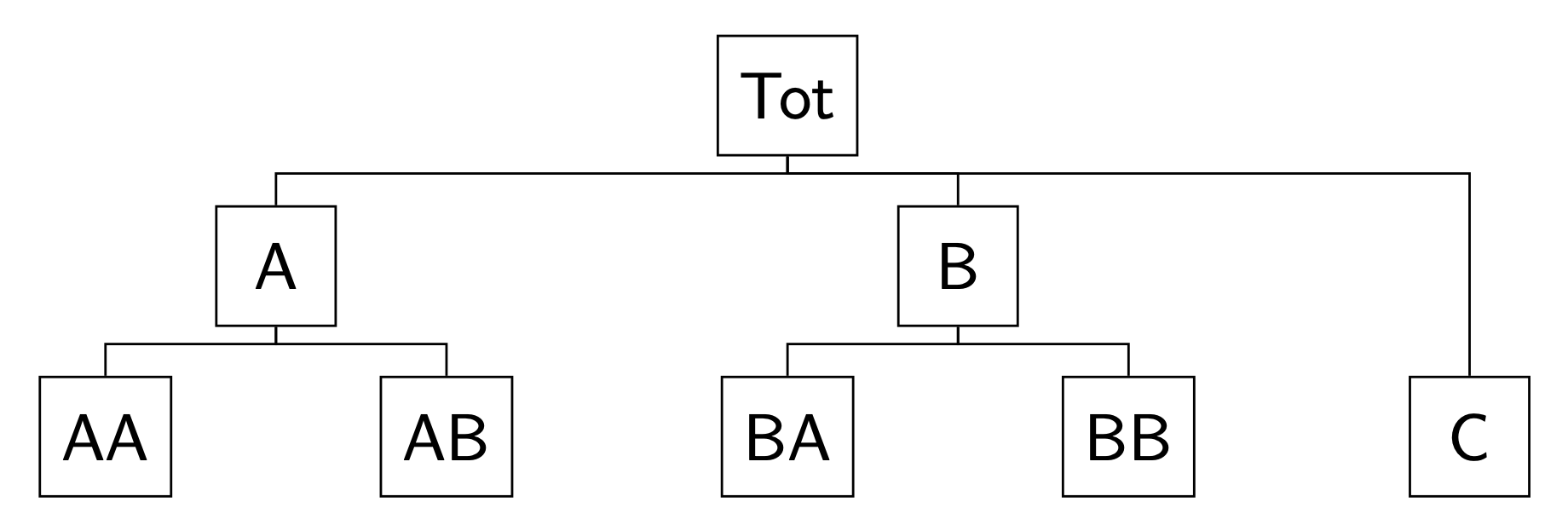Cross-sectional hierarchy

#### Simulation

In the following script we simulate five independent monthly bottom time series, each of length 180 (15 complete years of monthly data).

``````library(FoReco)
library(forecast)
library(sarima)
values <- NULL
base <- NULL
residuals <- NULL
test <- NULL

bottom <- matrix(NA, nrow = 180, ncol = 5)
# Model definition
bts <- list()
#ARIMA(1,0,0)(0,0,0)
bts[] <- list(ar=0.31,
nseasons=12)
#ARIMA(0,0,1)(0,0,0)
bts[] <- list(ma=0.61,
nseasons=12)
#ARIMA(0,1,1)(0,1,1)
bts[] <- list(ma=-0.1,
sma=-0.12,
iorder=1,
siorder=1,
nseasons=12)
#ARIMA(2,1,0)(0,0,0)
bts[] <- list(ar=c(0.38,0.25),
iorder=1,
nseasons=12)
#ARIMA(2,0,0)(0,1,1)
bts[] <- list(ar=c(0.30,0.12),
sma=0.23,
siorder=1,
nseasons=12)
mm <- c(58.85, 60.68, 59.26, 35.47, 58.61)
set.seed(525)
for(i in 1:5){
bottom[,i] <- mm[i] + sim_sarima(n=180, model = bts[[i]],
n.start = 200)
}
colnames(bottom) <- c("AA", "AB", "BA", "BB", "C")
C <- matrix(c(rep(1,5),
rep(1,2), rep(0,3),
rep(0,2), rep(1,2), 0), byrow = TRUE, nrow = 3)

upper <- bottom%*%t(C)
colnames(upper) <- c("T", "A", "B")
values\$k1 <- ts(cbind(upper, bottom), frequency = 12)
colnames(values\$k1) <- c("T", "A", "B", "AA", "AB", "BA", "BB", "C")``````

More precisely, AA is simulated from an AR(1) process, AB from an MA(1), BA from an ARIMA(0,1,1)(0,1,1), BB from an ARIMA(2,1,0), and C from an ARIMA(2,0,0)(0,1,1). The higher levels series in the hierarchy (T,A,B) are obtained by simple summation of the five bottom time series.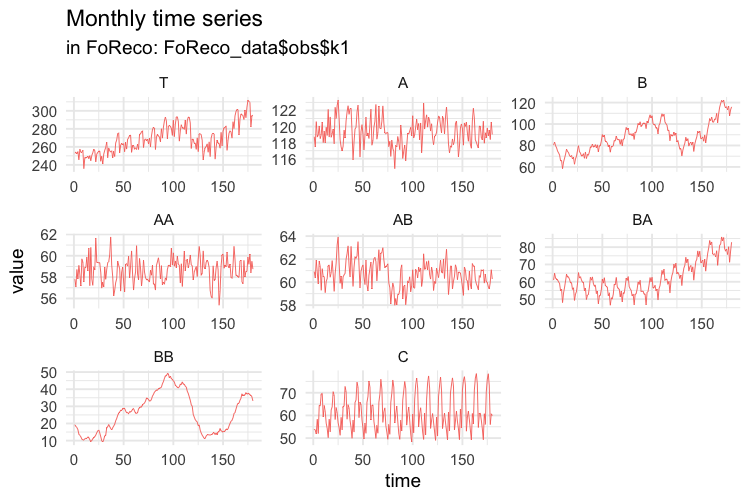Then we compute the temporally aggregated series at annual \((k = 12)\), semi-annual \((k = 6)\), four-monthly \((k = 4)\), quarterly \((k = 3)\), and bi-monthly \((k = 2)\) frequencies.

``````# BI-MONTHLY SERIES
values\$k2 <- ts(apply(values\$k1, 2,
function(x) colSums(matrix(x, nrow = 2))),
frequency = 6)``````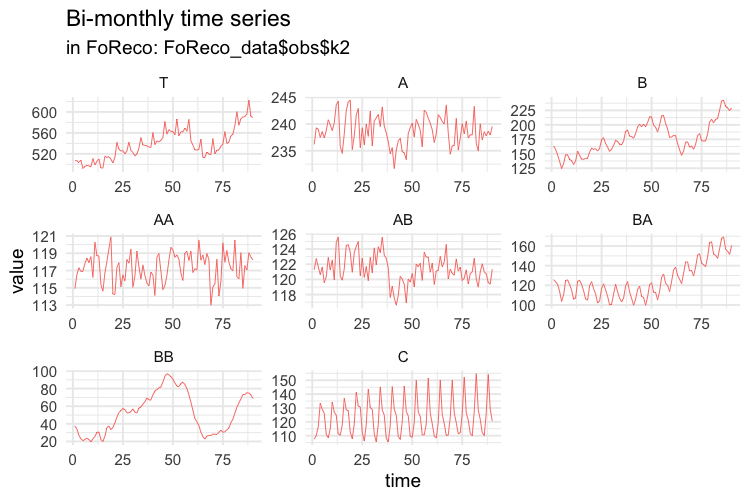``````# QUARTERLY SERIES
values\$k3 <- ts(apply(values\$k1, 2,
function(x) colSums(matrix(x, nrow = 3))),
frequency = 4)``````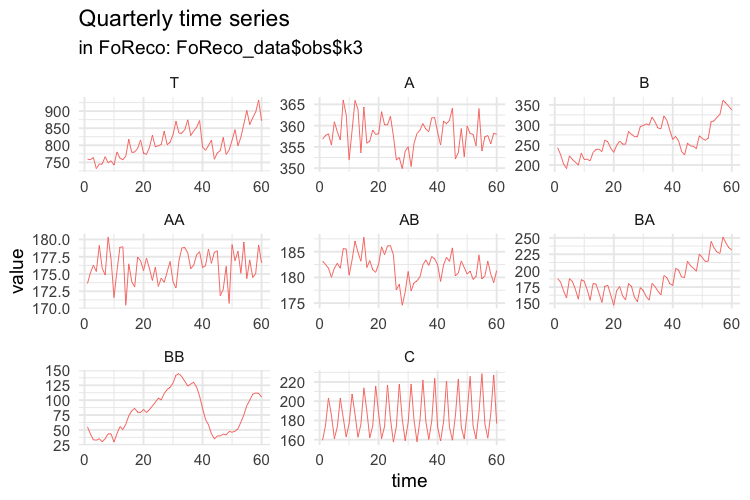``````# FOUR-MONTHLY SERIES
values\$k4 <- ts(apply(values\$k1, 2,
function(x) colSums(matrix(x, nrow = 4))),
frequency = 3)``````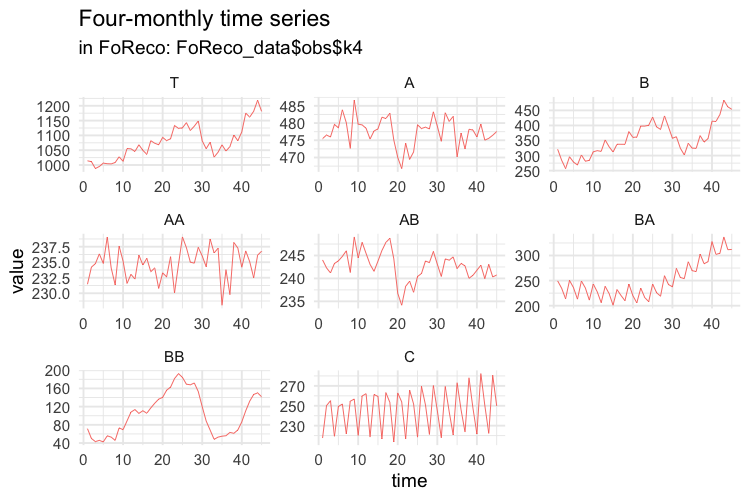``````# SEMI-ANNUAL SERIES
values\$k6 <- ts(apply(values\$k1, 2,
function(x) colSums(matrix(x, nrow = 6))),
frequency = 2)``````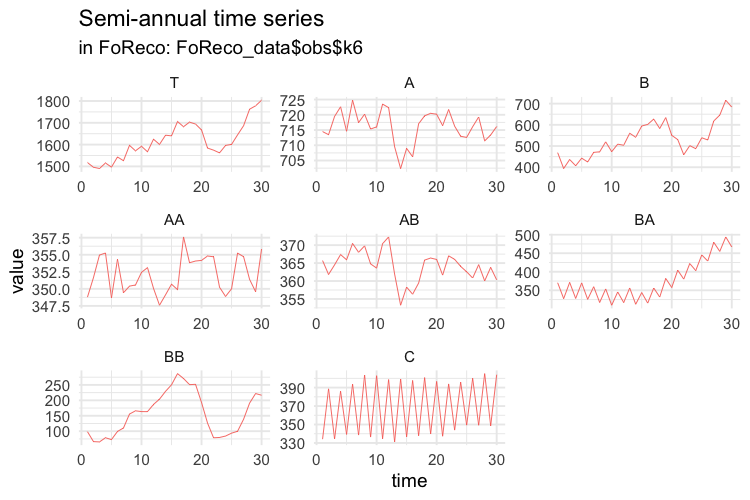``````# ANNUAL SERIES
values\$k12 <- ts(apply(values\$k1, 2,
function(x) colSums(matrix(x, nrow = 12))),
frequency = 1)``````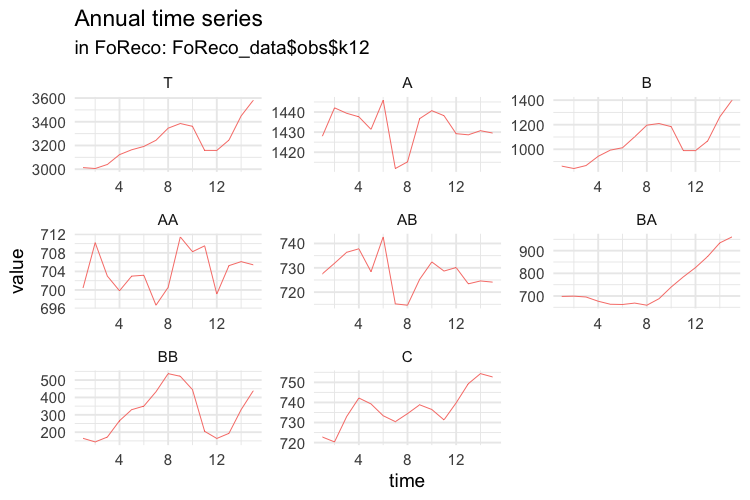The first 14 years of each simulated series are used as training set, and the last year as test set. The forecasts are obtained using the `auto.arima` function of the `forecast` package (Hyndman et al., 2020).

``````# MONTHLY FORECASTS
base\$k1 <- matrix(NA, nrow = 12, ncol = ncol(values\$k1))
residuals\$k1 <- matrix(NA, nrow = 168, ncol = ncol(values\$k1))
for (i in 1:ncol(values\$k1)) {
train <- values\$k1[1:168, i]
forecast_arima <- forecast(auto.arima(train), h = 12)
base\$k1[, i] <- forecast_arima\$mean
residuals\$k1[, i] <- forecast_arima\$residuals
}
base\$k1 <- ts(base\$k1, frequency = 12, start = c(15, 1))
colnames(base\$k1) <- c("T", "A", "B", "AA", "AB", "BA", "BB", "C")
residuals\$k1 <- ts(residuals\$k1, frequency = 12)
colnames(residuals\$k1) <- c("T", "A", "B", "AA", "AB", "BA", "BB", "C")
test\$k1 <- values\$k1[-c(1:168), ]``````

The following plots show the actual values and the forecasts for the test year at any temporal aggregation level.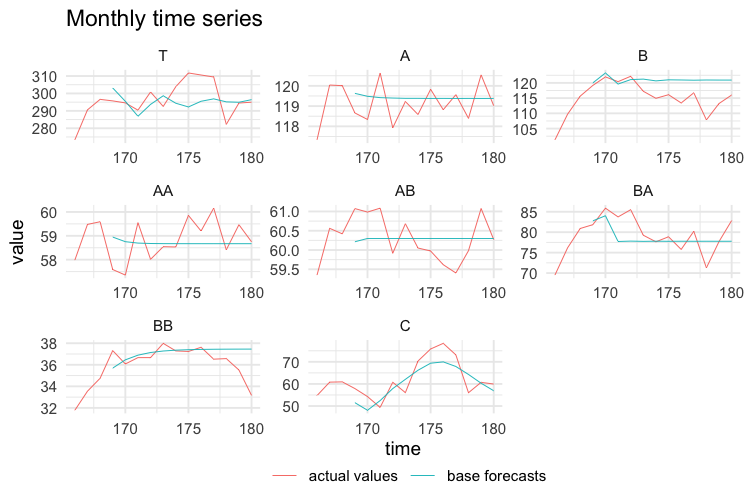``````# BI-MONTHLY FORECASTS
base\$k2 <- matrix(NA, nrow = 6, ncol = ncol(values\$k2))
residuals\$k2 <- matrix(NA, nrow = 84, ncol = ncol(values\$k2))
for (i in 1:ncol(values\$k2)) {
train <- values\$k2[1:84, i]
forecast_arima <- forecast(auto.arima(train), h = 6)
base\$k2[, i] <- forecast_arima\$mean
residuals\$k2[, i] <- forecast_arima\$residuals
}
base\$k2 <- ts(base\$k2, frequency = 6, start = c(15, 1))
colnames(base\$k2) <- c("T", "A", "B", "AA", "AB", "BA", "BB", "C")
residuals\$k2 <- ts(residuals\$k2, frequency = 6)
colnames(residuals\$k2) <- c("T", "A", "B", "AA", "AB", "BA", "BB", "C")
test\$k2 <- values\$k2[-c(1:84), ]``````